# Strand7 nonlinear static solver

Although the majority of engineering structures operate within the linear regime and the assumptions made for the linear solution are valid, there is however a wide class of problems which do exhibit nonlinear behaviour. The nonlinear static solver predicts the response of structures with such nonlinear behaviour taken into account. In Strand7, three types of nonlinearities can be included: geometric, material and boundary nonlinearity.

The nonlinear static solver performs the following steps:
• Initialises the nodal displacement vector, element stress, strain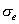,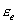etc.

• Sets the current load increment.

• Calculates and assembles the element stiffness matrices (if required), equivalent element force vectors and external nodal force vectors. In the stiffness calculation, material temperature dependency is considered. Depending on whether material and/or geometric nonlinearity is considered, the current material modulus and geometry will be used. The element geometric stiffness matrix is also included if the corresponding option is set. Either consistent or lumped element equivalent load vectors can be calculated according to the option setting. Constraints are also assembled in this process and the constant terms in the enforced displacements and shrink links are combined and applied.

At the end of this assembly procedure, the following linear equation system of equilibrium is formed: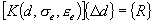where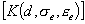- Current global stiffness matrix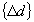- Displacement increment vector- Global residual force vector or unbalanced force vector with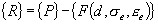in which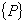and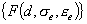are current external force and element nodal force vectors, respectively.

• Solves the above equation for• Updates the total nodal displacement vector

• Checks convergence

displacement norm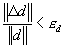residual force norm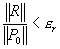where

•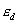and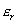are convergence tolerance on displacement and residual force,and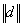are norms of incremental and total displacement vectors,is the norm of the residual force vector at the first iteration of each time step andis the norm of the residual force vector in the current iteration.

• If both of the convergence criteria are satisfied, start the next load step or stop at the last load step. If either of the criteria is not satisfied, continue the iteration.

### Notes

The Strand7 nonlinear static solver uses an algorithm based on modified Newton-Raphson method. Major features of this algorithm are:

1. The load increments are defined in the load increments table. In this table, each column contains load combination factors for the corresponding load steps or increments. For example, if the full load is applied in ten steps, there will be ten columns in this table. In each column, combination factors are required for the pre-defined basic load cases to determine the amount of the load from each load case to be applied in the corresponding step.

2. Multiple freedom cases may be used, with the constant terms combined using the factors in the load increment table.

3. The stiffness matrix need not be updated in every iteration. Different strategies for global stiffness matrix updating are available:

1. Update at every iteration.
2. Update only in the first iteration of a load step.
3. Update only in the first two iterations of a load step.
4. Let the solver decide whether to update or not, based on the following rules:
1. Displacement change.
2. Maximum number of iterations for no stiffness update.

4. Automatic load step adjustment can be enabled to control the load increment to achieve a better convergence rate and more importantly avoid divergence. Before the solution is available, it is difficult to know exactly how the load steps should be set. By using the automatic load stepping, the solver will adjust the load based on the displacement and any divergence tendency in the solution.

5. Contact elements are considered irrespective of the type of nonlinearity chosen in the solver panel.

6. Restart can be used to continue a previously completed or aborted solution. The restart can proceed from any of the previously saved solution steps, provided the restart file has been saved.

For more information on nonlinear static analysis, see Strand7 Webnotes - Nonlinear / Statics.